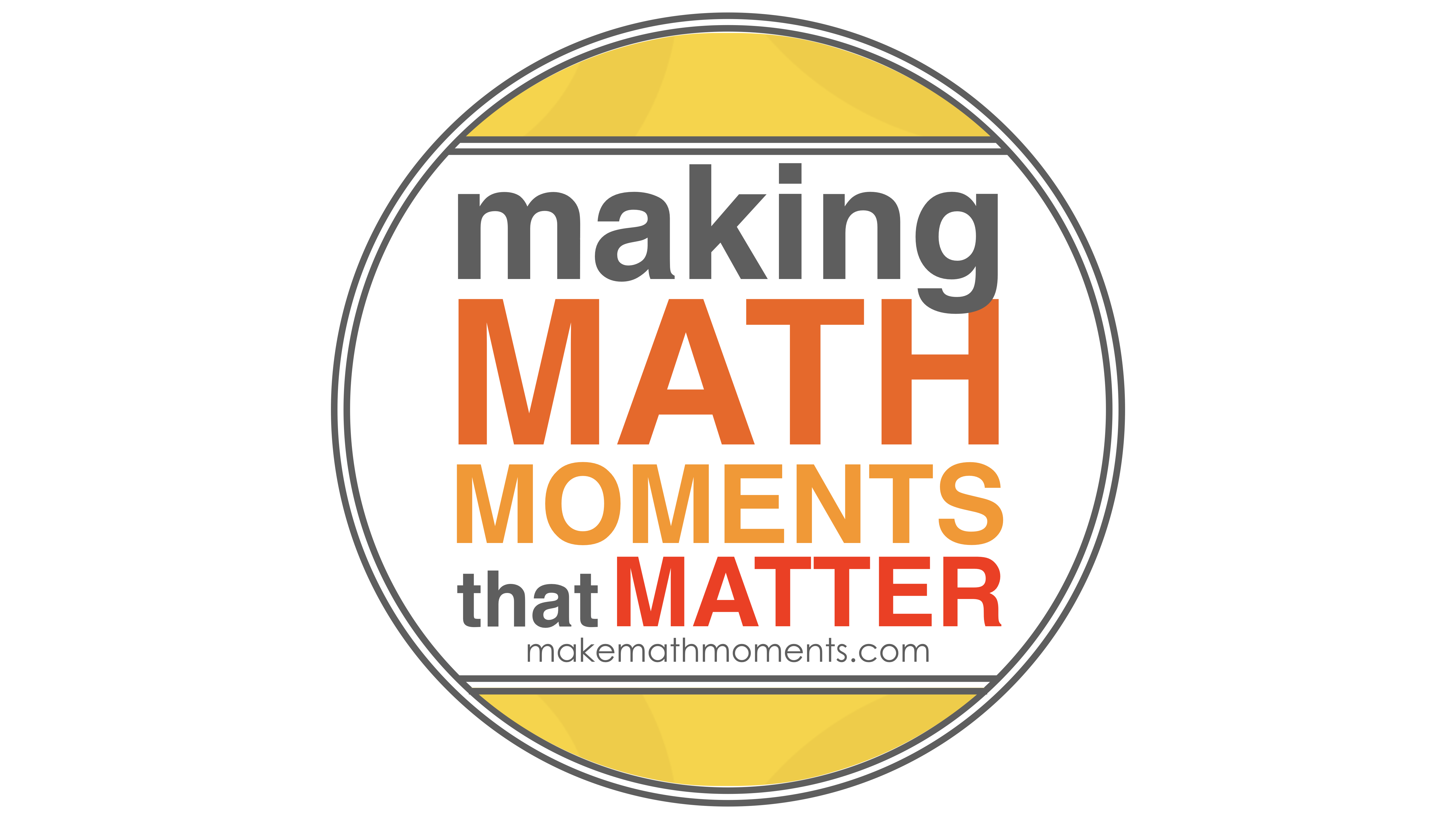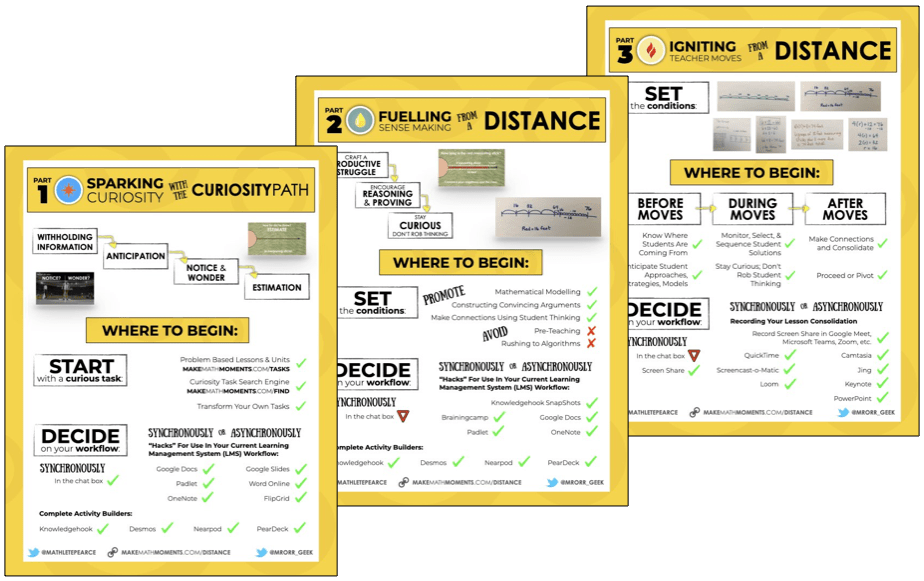# Category - Measurement

## Understanding Area of a Triangle Conceptually

Let's tackle Area of a Triangle conceptually using a series of visual prompts to help students generate the formula for finding the area of ANY...

## How To Convert Units of Measurement Visually

Tackle Measurement and in particular unit conversion conceptually using a series of visual prompts to help students generate relationships between...

## Understanding Area of a Rectangle Conceptually

Let's tackle Area of a Rectangle conceptually using a series of visual prompts to help students generate the formula for finding the area of ANY...